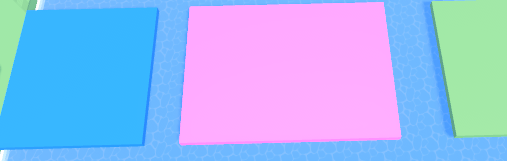# Position new part with different size, but gap is staying the smae

Im trying to make path and each time new part will be summoning it will be 1.5 bigger than a last one.

here is my script:

``````local temp = script.Parent.Part

local times = 1

local function colorPart()
if times == 1 then
temp.BrickColor = BrickColor.new("Pink")
elseif times == 2 then
temp.BrickColor = BrickColor.new("Shamrock")
elseif times == 3 then
temp.BrickColor = BrickColor.new("Daisy orange")
elseif times == 4 then
temp.BrickColor = BrickColor.new("Bright blue")
times = 1
end
end

for i=1,50 do
local newTemp = temp:Clone()
newTemp.Parent = script.Parent
newTemp.Size = Vector3.new(temp.Size.X*1.5,temp.Size.Y,temp.Size.Z)-- this line positiong is wrong
newTemp.Position = Vector3.new(temp.Position.X,temp.Position.Y,temp.Position.Z+newTemp.Size.X)
temp = newTemp
colorPart()
times += 1
wait()
end
``````

Once i run it it will look like this:problem is with positiong it right, i want no gap between, like this:``````local temp = script.Parent.Part

local times = 1

local function colorPart()
if times == 1 then
temp.BrickColor = BrickColor.new("Pink")
elseif times == 2 then
temp.BrickColor = BrickColor.new("Shamrock")
elseif times == 3 then
temp.BrickColor = BrickColor.new("Daisy orange")
elseif times == 4 then
temp.BrickColor = BrickColor.new("Bright blue")
times = 1
end
end

for i=1,50 do
local newTemp = temp:Clone()
newTemp.Parent = script.Parent
newTemp.Size = Vector3.new(temp.Size.X*1.5,temp.Size.Y,temp.Size.Z)-- this line positiong is wrong
newTemp.Position = Vector3.new(temp.Position.X,temp.Position.Y,temp.Position.Z+newTemp.Size.X / 2 + temp.Size.X / 2)
temp = newTemp
colorPart()
times += 1
wait()
end
``````
1 Like

This topic was automatically closed 14 days after the last reply. New replies are no longer allowed.